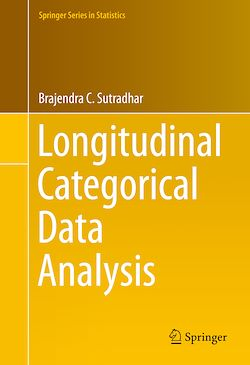# Longitudinal Categorical Data Analysis

-English
369 Pages

Description

This is the first book in longitudinal categorical data analysis with parametric correlation models developed based on dynamic relationships among repeated categorical responses. This book is a natural generalization of the longitudinal binary data analysis to the multinomial data setup with more than two categories. Thus, unlike the existing books on cross-sectional categorical data analysis using log linear models, this book uses multinomial probability models both in cross-sectional and longitudinal setups. A theoretical foundation is provided for the analysis of univariate multinomial responses, by developing models systematically for the cases with no covariates as well as categorical covariates, both in cross-sectional and longitudinal setups. In the longitudinal setup, both stationary and non-stationary covariates are considered. These models have also been extended to the bivariate multinomial setup along with suitable covariates. For the inferences, the book uses the generalized quasi-likelihood as well as the exact likelihood approaches.

The book is technically rigorous, and, it also presents illustrations of the statistical analysis of various real life data involving univariate multinomial responses both in cross-sectional and longitudinal setups. This book is written mainly for the graduate students and researchers in statistics and social sciences, among other applied statistics research areas. However, the rest of the book, specifically the chapters from 1 to 3, may also be used for a senior undergraduate course in statistics.

Subjects

##### Biostatistics

Informations